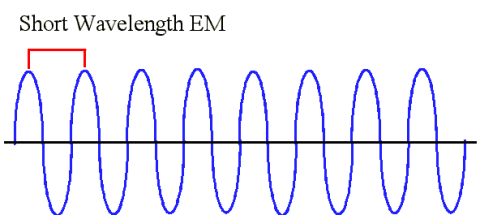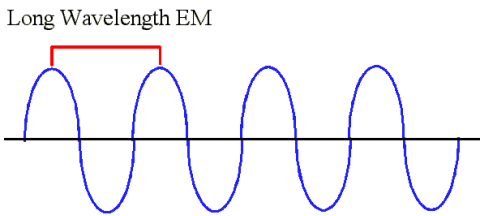Electromagnetic radiation is a type of energy that is commonly known as light. Generally speaking, we say that light travels in waves, and all electromagnetic radiation travels at the same speed which is about 3.0 * 108 meters per second through a vacuum. We call this the "speed of light"; nothing can move faster than the speed of light.A wavelength is one cycle of a wave, and we measure it as the distance between any two consecutive peaks of a wave. The peak is the highest point of the wave, and the trough is the lowest point of the wave.

## Frequency

So when we talk about light traveling in waves, we can also talk about frequency, or the number of wavelengths that pass a certain point in a given length of time. We usually measure this as the number of wavelength cycles that pass per second. The units for this measurement are Hertz (hz).

So, if the wavelength of a light wave is shorter, that means that the frequency will be higher because one cycle can pass in a shorter amount of time. This means that more cycles can pass by the set point in 1 second. Likewise, a light wave that has a longer wavelength will have a lower frequency because each cycle takes a longer time to complete.This is an example of a shorter wavelength. Like we said earlier, if you were an observer standing on the black line, and you were so quick that you could count the number of wave peaks that passed you in one second, you would find that the number of wave peaks that you counted would be higher than a longer wavelength. This is an example of a longer wavelength. Suppose you put yourself on the line again and you start clocking the number of wave peaks that pass you. Wait a minute, not nearly so many wave peaks have gone by in one second like before with the shorter wavelength. That means that longer wavelengths have a lower frequency.

Conclusion: a longer wavelength means a lower frequency, and a shorter wavelength means a higher frequency!

## How does this relate to the Electromagnetic Spectrum?

Like we said before, light can be characterized by its wavelength. For example, the human eye can detect light at wavelengths between 400 to 900 nanometers. That is why we call this part of the electromagnetic spectrum visible light. Light waves with shorter wavelengths (and higher frequencies) also have more energy, so types of light like gamma rays, X-rays, and ultraviolet light are more energetic than visible light, and infrared, microwaves, and radio waves are less energetic than the light in the visible spectrum.

To go back to the electromagnetic spectrum.